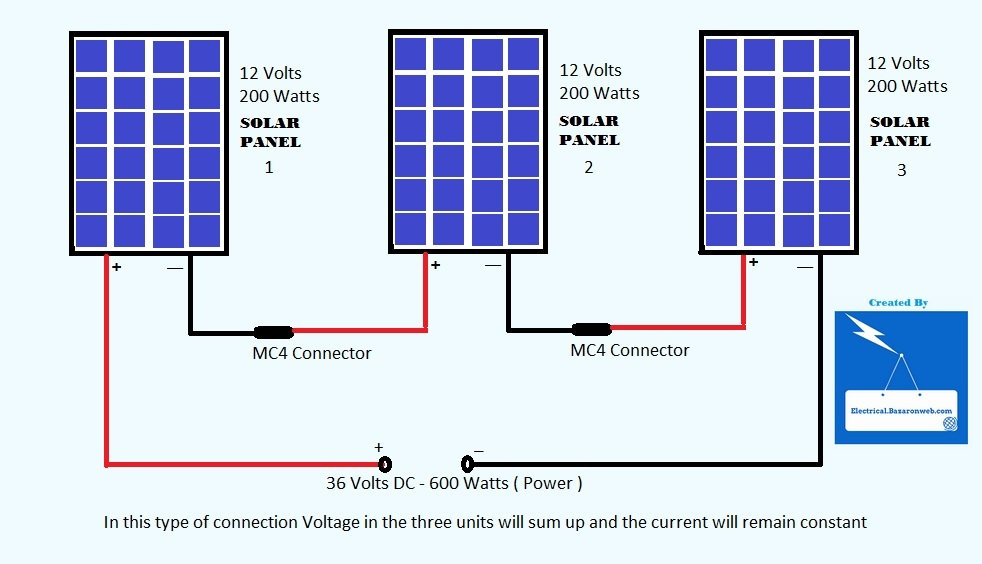September 16, 2015# How to Connect Solar Panels in Series

#### How to Connect Solar Panels in Series

Hello Folks, This is Ankit from Electrical Bazar on web or you can spell it Electrical Bazaar on web as per your liking.

The motive of this post is to demonstrate how to connect Solar Panels of Same rating in series connection. In a series connection “Current Remains the same” and the voltage of Units is mathematically added. Simply refer to this diagram and the concept will be clear to you.

In this diagram we have taken an example of Solar Panels with Same Rating of Voltage and Power. Since every module is of 12 Volts and there are 3 Units,  the output is 12 x 3 = 36 Volts and the total Output Power is 200 x 3 = 600 Watts. This type of connection is favorable when the rated voltage of Inverter / Household circuit is of 36 Volts.

The above arrangement can be converted into a 24 Volts System and a 12 Volts System using different Series – Parallel combinations. We will upload them later.

Questions ?

1.bern says: